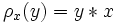# Loop

Jump to: navigation, search
This is a variation of group|Find other variations of group | Read a survey article on varying group
This article defines a property that can be evaluated for a magma, and is invariant under isomorphisms of magmas.
View other such properties
QUICK PHRASES: group without associativity, unital magma with unique left and right quotients

## Definition

An algebra loop, sometimes simply called a loop, is defined as a set$L$ equipped with a binary operation$*$, such that the following are true:

• neutral element: There exists an element$e \in L$ such that$a * e = e * a = a$$\forall a \in L$. Such an$e$ is termed a neutral element (or identity element or unit).
• For any$a,b \in L$ there exist unique solutions$x,y \in L$ to the equation:$a * x = y * a = b$

Equivalently, an algebra loop is a quasigroup with a neutral element (also called identity element or unit).

## Relation with other structures

### Stronger structures

Structure Meaning Proof of implication Proof of strictness (reverse implication failure) Intermediate notions
group associative loop not implies group Alternative loop, Power-associative loop|FULL LIST, MORE INFO
Moufang loop Alternative loop, Power-associative loop|FULL LIST, MORE INFO
Alternative loop |FULL LIST, MORE INFO

For more, refer Category:Loop properties.

## Groups associated to an algebra loop

### Left multiplication group

For an algebra loop$L$, every element$x \in L$ defines a permutation$\lambda_x$ on$L$ by$\lambda_x(y) = x * y$. The group generated by all these permutations is termed the left multiplication group. Note that the composite of$\lambda_x$ and$\lambda_y$ is not necessarily$\lambda_{x * y}$ because we are not assuming associativity.

Note that when$L$ is a group, the mapping$x \mapsto \lambda_x$ defines an isomorphism of groups, hence$L$ is isomorphic to its left multiplication group. Further, the action of$L$ (as the left multiplication group) on itself is the left regular representation.

### Right multiplication group

For an algebra loop$L$, every element$x \in L$ defines a permutation$\rho_x$ on$L$ by$\rho_x(y) = y * x$. The group generated by all these permutations is termed the right multiplication group.

Note that when$L$ is a group, the mapping$x \mapsto \rho_x$ defines an anti-isomorphism of groups, hence$L$ is isomorphic to its right multiplication group. Further, the action of$L$ (as the right multiplication group) on itself is the right regular representation.

### Left inner mapping group

The left inner mapping group for an algebra loop is the subgroup of the left multiplication group, comprising those elements that send the identity element to itself.

When the algebra loop is a group, the left inner mapping group is the trivial subgroup.

### Right inner mapping group

The right inner mapping group for an algebra loop is the subgroup of the right multiplication group, comprising those elements that send the identity element (of the loop) to itself.